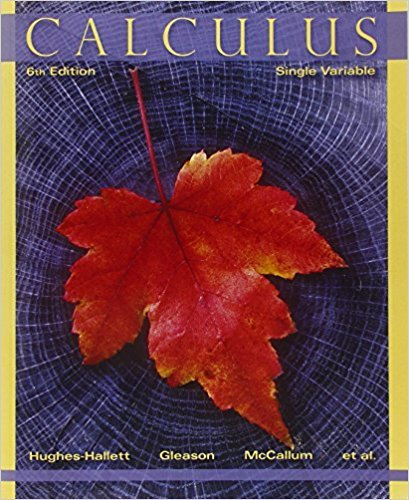×
×

# Solutions for Chapter 3.7: THE CHAIN RULE AND INVERSE FUNCTIONS## Full solutions for Calculus: Single Variable | 6th Edition

ISBN: 9780470888643Solutions for Chapter 3.7: THE CHAIN RULE AND INVERSE FUNCTIONS

Solutions for Chapter 3.7
4 5 0 335 Reviews
27
5
##### ISBN: 9780470888643

Chapter 3.7: THE CHAIN RULE AND INVERSE FUNCTIONS includes 1 full step-by-step solutions. This expansive textbook survival guide covers the following chapters and their solutions. This textbook survival guide was created for the textbook: Calculus: Single Variable , edition: 6. Calculus: Single Variable was written by and is associated to the ISBN: 9780470888643. Since 1 problems in chapter 3.7: THE CHAIN RULE AND INVERSE FUNCTIONS have been answered, more than 33454 students have viewed full step-by-step solutions from this chapter.

Key Calculus Terms and definitions covered in this textbook
• Annual percentage rate (APR)

The annual interest rate

• Binomial theorem

A theorem that gives an expansion formula for (a + b)n

• Blocking

A feature of some experimental designs that controls for potential differences between subject groups by applying treatments randomly within homogeneous blocks of subjects

• Boundary

The set of points on the “edge” of a region

• Bounded interval

An interval that has finite length (does not extend to ? or -?)

• Ellipse

The set of all points in the plane such that the sum of the distances from a pair of fixed points (the foci) is a constant

• Gaussian elimination

A method of solving a system of n linear equations in n unknowns.

• Half-angle identity

Identity involving a trigonometric function of u/2.

• Integers

The numbers . . ., -3, -2, -1, 0,1,2,...2

• Inverse secant function

The function y = sec-1 x

• Lemniscate

A graph of a polar equation of the form r2 = a2 sin 2u or r 2 = a2 cos 2u.

• Lower bound test for real zeros

A test for finding a lower bound for the real zeros of a polynomial

• Numerical model

A model determined by analyzing numbers or data in order to gain insight into a phenomenon, p. 64.

• Odd function

A function whose graph is symmetric about the origin (ƒ(-x) = -ƒ(x) for all x in the domain of f).

• Quotient polynomial

See Division algorithm for polynomials.

• Randomization

The principle of experimental design that makes it possible to use the laws of probability when making inferences.

• Riemann sum

A sum where the interval is divided into n subintervals of equal length and is in the ith subinterval.

• Vertex of a parabola

The point of intersection of a parabola and its line of symmetry.

• Wrapping function

The function that associates points on the unit circle with points on the real number line

• Xscl

The scale of the tick marks on the x-axis in a viewing window.

×